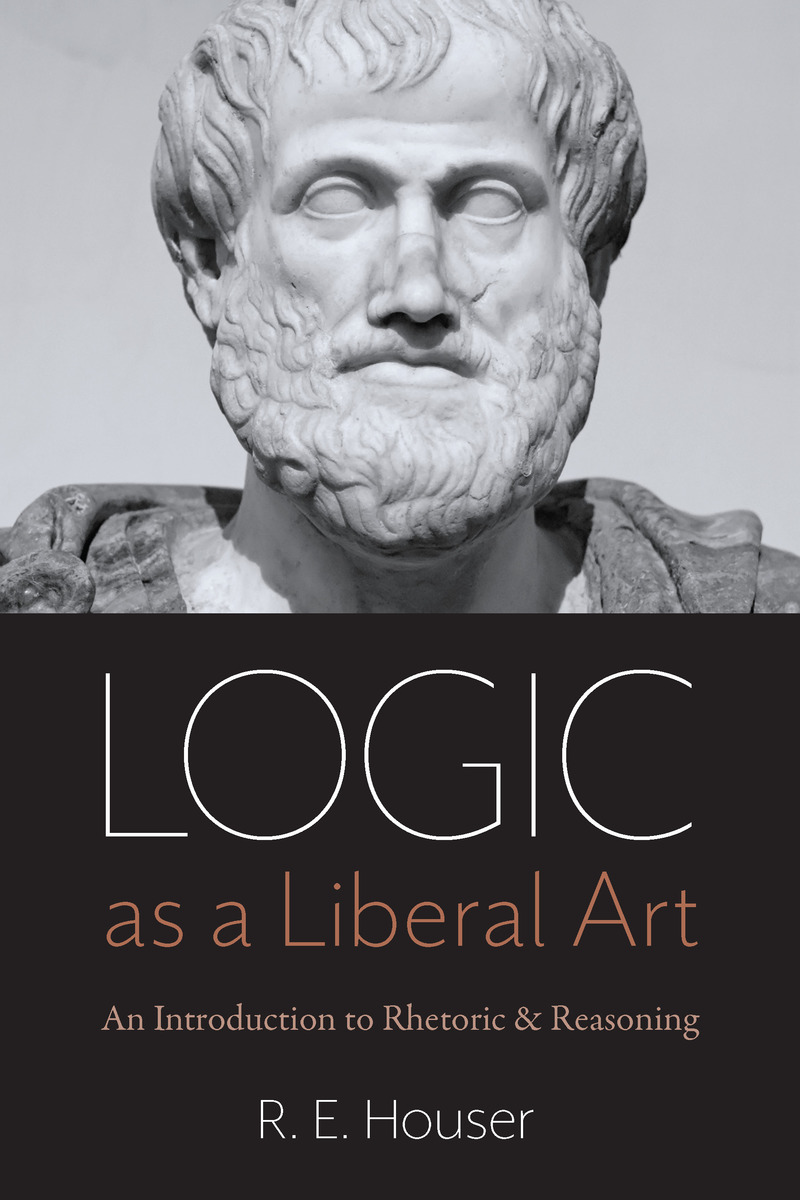## Logic as a Liberal Art: An Introduction to Rhetoric and Reasoning

##### eISBN: 978-0-8132-3235-5, Paper: 978-0-8132-3234-8

In the twenty-first century there are two ways to study logic. The more recent approach is symbolic logic. The history of teaching logic since World War II, however, casts doubt on the idea that symbolic logic is best for a first logic course. Logic as a Liberal Art is designed as part of a minority approach, teaching logic in the "verbal" way, in the student's "natural" language, the approach invented by Aristotle. On utilitarian grounds alone, this "verbal" approach is superior for a first course in logic, for the whole range of students.

For millennia, this "verbal" approach to logic was taught in conjunction with grammar and rhetoric, christened the trivium. The decline in teaching grammar and rhetoric in American secondary schools has led Dr. Rollen Edward Houser to develop this book. The first part treats grammar, rhetoric, and the essential nature of logic. Those teachers who look down upon rhetoric are free, of course, to skip those lessons. The treatment of logic itself follows Aristotle's division of the three acts of the mind (Prior Analytics 1.1). Formal logic is then taken up in Aristotle's order, with Parts on the logic of Terms, Propositions, and Arguments.

The emphasis in Logic as a Liberal Art is on learning logic through doing problems. Consequently, there are more problems in each lesson than would be found, for example, in many textbooks. In addition, a special effort has been made to have easy, medium, and difficult problems in each Problem Set. In this way the problem sets are designed to offer a challenge to all students, from those most in need of a logic course to the very best students.
Contents
Acknowledgments
A Note to the Logic Instructor
A Note to the Student
Part 1. Grammar, Rhetoric, and Logic
Lesson 1. A Grounding in Grammar
Problem Set 1: Grammar Review
Lesson 2. Rhetoric: An Introduction
Problem Set 2: Rhetorical Analysis
Lesson 3. The Canon of Five Rhetorical Skills and the Five Parts of a Classical Speech
Problem Set 3: The Rhetoric of Socrates in the Apology
Lesson 4. The Three Rhetorical Appeals
Problem Set 4: The Three Appeals
Lesson 5. Aristotle Invents Logic—Twice
Problem Set 5: Problem Solving
Lesson 6. Aristotle Organizes the Logic of Discovery and Proof
Problem Set 6: Three Acts of the Mind
Part 2. The Logic of Terms
Lesson 7. Language, Thought, and Reality
Problem Set 7: Signs
Lesson 8. Categories: Working toward Definitions by Answering the “What?” Question
Problem Set 8: Recognizing and Using Categories
Lesson 9. Clarifying Concepts through Division and Collection of Terms
Problem Set 9: Argument Using Division
Lesson 10. Aristotle’s Predicables
Problem Set 10: Recognizing Essence, Property, and Accident
Lesson 11. Answering the “Why?” Question: Causes
Problem Set 11: The Four Causes
Lesson 12. Different Kinds of Definitions
Problem Set 12: Definitions
Part 3. The Logic of Propositions
Lesson 13. Statements and Propositions
Problem Set 13: Statements and Propositions
Lesson 14. Properties of Categorical Propositions
Problem Set 14: Basic Categorical Propositions
Lesson 15. Recognizing the Kinds of Categorical Propositions
Problem Set 15: Advanced Categorical Propositions
Lesson 16. Categorical Propositions in Context
Problem Set 16: Propositions in Context
Lesson 17. Euler and Venn Diagrams of Propositions
Problem Set 17: Euler and Venn Diagrams of Propositions
Lesson 18. Opposition
Problem Set 18: Opposition
Lesson 19. Conversion
Problem Set 19: Conversion
Lesson 20. Obversion
Problem Set 20: Obversion and Advanced Manipulations
Lesson 21. Hypothetical Propositions
Problem Set 21: Hypothetical Propositions
Problem Set 22: Advanced Hypothetical Propositions
Part 4. The Logic of Arguments
Lesson 23. Two Kinds of Reasoning
Problem Set 23: Distinguishing Deductive from Inductive Reasoning
Lesson 24. The Categorical Syllogism
Problem Set 24: Identifying Categorical Syllogisms
Lesson 25. Validity of Categorical Syllogisms
Problem Set 25: Validity of Categorical Syllogisms
Lesson 26. Categorical Syllogisms in Prose
Problem Set 26: Categorical Syllogisms in Prose
Lesson 27. Venn Diagrams of Categorical Syllogisms
Problem Set 27: Venn Diagrams of Categorical Syllogisms
Lesson 28. Enthymemes and Epicheiremas
Problem Set 28: Enthymemes and Epicheiremas
Lesson 29. Extended Categorical Arguments
Problem Set 29: Longer Categorical Arguments
Lesson 30. Hypothetical Arguments
Problem Set 30: Hypothetical Arguments